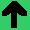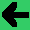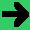# Matrices to Represent Graphs

• One way to represent a graph is by an adjacency matrix.
• If there are E nodes, make an ExE table.
• If there is a connection from Node x to Node y, put a 1 in row x column y, if not put in a zero.
• For undirected graphs, you only need a triangle.
• Exercise. There is a graph with 4 nodes, N0 is connected to all nodes, N1 and N3 to each other, and N2 to everything but N0.